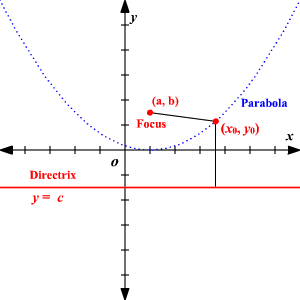# Finding the Equation of a Parabola Given Focus and Directrix

Given the focus and directrix of a parabola , how do we find the equation of the parabola?

If we consider only parabolas that open upwards or downwards, then the directrix will be a horizontal line of the form $y=c$ .

Let $\left(a,b\right)$ be the focus and let $y=c$ be the directrix. Let $\left({x}_{0},{y}_{0}\right)$ be any point on the parabola.Any point, $\left({x}_{0},{y}_{0}\right)$ on the parabola satisfies the definition of parabola, so there are two distances to calculate:

1. Distance between the point on the parabola to the focus
2. Distance between the point on the parabola to the directrix

To find the equation of the parabola, equate these two expressions and solve for ${y}_{0}$ .

Find the equation of the parabola in the example above.

Distance between the point $\left({x}_{0},{y}_{0}\right)$ and $\left(a,b\right)$ :

$\sqrt{{\left({x}_{0}-a\right)}^{2}+{\left({y}_{0}-b\right)}^{2}}$

Distance between point $\left({x}_{0},{y}_{0}\right)$ and the line $y=c$ :

$|\text{\hspace{0.17em}}{y}_{0}-c\text{\hspace{0.17em}}|$

(Here, the distance between the point and horizontal line is difference of their $y$ -coordinates.)

Equate the two expressions.

$\sqrt{{\left({x}_{0}-a\right)}^{2}+{\left({y}_{0}-b\right)}^{2}}=|\text{\hspace{0.17em}}{y}_{0}-c\text{\hspace{0.17em}}|$

Square both sides.

${\left({x}_{0}-a\right)}^{2}+{\left({y}_{0}-b\right)}^{2}={\left({y}_{0}-c\right)}^{2}$

Expand the expression in ${y}_{0}$ on both sides and simplify.

${\left({x}_{0}-a\right)}^{2}+{b}^{2}-{c}^{2}=2\left(b-c\right){y}_{0}$

This equation in $\left({x}_{0},{y}_{0}\right)$ is true for all other values on the parabola and hence we can rewrite with $\left(x,y\right)$ .

Therefore, the equation of the parabola with focus $\left(a,b\right)$ and directrix $y=c$ is

${\left(x-a\right)}^{2}+{b}^{2}-{c}^{2}=2\left(b-c\right)y$

Example:

If the focus of a parabola is $\left(2,5\right)$ and the directrix is $y=3$ , find the equation of the parabola.

Let $\left({x}_{0},{y}_{0}\right)$ be any point on the parabola. Find the distance between $\left({x}_{0},{y}_{0}\right)$ and the focus. Then find the distance between $\left({x}_{0},{y}_{0}\right)$ and directrix. Equate these two distance equations and the simplified equation in ${x}_{0}$ and ${y}_{0}$ is equation of the parabola.

The distance between $\left({x}_{0},{y}_{0}\right)$ and $\left(2,5\right)$ is $\sqrt{{\left({x}_{0}-2\right)}^{2}+{\left({y}_{0}-5\right)}^{2}}$

The distance between $\left({x}_{0},{y}_{0}\right)$ and the directrix, $y=3$ is

$|\text{\hspace{0.17em}}{y}_{0}-3\text{\hspace{0.17em}}|$ .

Equate the two distance expressions and square on both sides.

$\sqrt{{\left({x}_{0}-2\right)}^{2}+{\left({y}_{0}-5\right)}^{2}}=|\text{\hspace{0.17em}}{y}_{0}-3\text{\hspace{0.17em}}|$

${\left({x}_{0}-2\right)}^{2}+{\left({y}_{0}-5\right)}^{2}={\left({y}_{0}-3\right)}^{2}$

Simplify and bring all terms to one side:

${x}_{0}{}^{2}-4{x}_{0}-4{y}_{0}+20=0$

Write the equation with ${y}_{0}$ on one side:

${y}_{0}=\frac{{x}_{0}{}^{2}}{4}-{x}_{0}+5$

This equation in $\left({x}_{0},{y}_{0}\right)$ is true for all other values on the parabola and hence we can rewrite with $\left(x,y\right)$ .

So, the equation of the parabola with focus $\left(2,5\right)$ and directrix is $y=3$ is

$y=\frac{{x}^{2}}{4}-x+5$